﻿ Faraday's Law of Induction Experiments for Lesson Plans & Science Fair Projects
Faraday's Law of Induction
K-12 Experiments & Background Information
For Science Labs, Lesson Plans, Class Activities, Homework Help & Science Fair Projects
For Middle and High School Students and Teachers

Faraday's Law of Induction Experiments

Faraday's Law of Induction

## Definition

Faraday's law of induction (or the law of electromagnetic induction) states that the induced electromotive force in a closed loop is directly proportional to the time rate of change of magnetic flux through the loop.

## Basics

Moving a conductor (such as a metal wire) through a magnetic field produces a voltage in that conductor. The resulting voltage is proportional to the speed of movement: moving the conductor twice as fast produces twice the voltage. The magnetic field, the direction of movement, and the voltage are all at right angles to each other. Whenever movement creates voltage, Fleming's right hand rule describes the direction of the voltage. A fixed conductor will also have an induced voltage if the magnetic flux in the area enclosed by the conductor is changing.

For the common but special case of a coil of wire, composed of N loops with the same area, Faraday's law of electromagnetic induction states that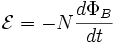where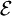is the electromotive force (emf) in volts
N is the number of turns of wire
ΦB is the magnetic flux in webers through a single loop. The direction of the electromotive force (the negative sign in the above formula) was first given by Lenz's law.

More generally, the relation between the rate of change of the magnetic flux through the surface S enclosed by a contour C and the electric field along the contour is defined as: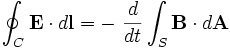where

E is the electric field,
dl is an infinitesimal element of the contour C,
B is the magnetic field.

The directions of the contour C and of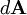are assumed to be related by the right-hand rule.

Equivalently, the differential form of Faraday's law is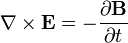which is one of the Maxwell equations.

This principle is used for measuring the flow of electrically conductive liquids and slurries. Such instruments are called Magnetic Flow Meters. The induced voltage U generated in the magnetic field B due to a conductive liquid moving at velocity v is thus given by: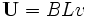$\mathbf U= BLv$,

where L is the distance between electrodes in the magnetic flow meter.

Faraday's law, along with the other laws of electromagnetism, was later incorporated into Maxwell's equations, unifying all of electromagnetism.

Faraday's law of induction is based on Michael Faraday's experiments in 1831. The effect was also discovered by Joseph Henry at about the same time, but Faraday published first.

Lenz's law gives the direction of the induced electromotive force (emf) and current resulting from electromagnetic induction. German physicist Heinrich Lenz formulated it in 1834

## Topics of Interest

### Faraday's law as two different phenomena

Some physicists have remarked that Faraday's law is a single equation describing two different phenomena: The motional EMF generated by a magnetic force on a moving wire, and the transformer EMF generated by an electric force due to a changing magnetic field. James Clerk Maxwell drew attention to this fact in his 1861 paper On Physical Lines of Force. In the latter half of part II of that paper, Maxwell gives a separate physical explanation for each of the two phenomena. A reference to these two aspects of electromagnetic induction is made in some modern textbooks. As Richard Feynman states:

So the "flux rule" that the emf in a circuit is equal to the rate of change of the magnetic flux through the circuit applies whether the flux changes because the field changes or because the circuit moves (or both).... Yet in our explanation of the rule we have used two completely distinct laws for the two cases – the first for "circuit moves" and the second for "field changes".
We know of no other place in physics where such a simple and accurate general principle requires for its real understanding an analysis in terms of two different phenomena.

Richard P. Feynman,   The Feynman Lectures on Physics

Reflection on this apparent dichotomy was one of the principal paths that led Einstein to develop special relativity:

It is known that Maxwell’s electrodynamics—as usually understood at the present time—when applied to moving bodies, leads to asymmetries which do not appear to be inherent in the phenomena. Take, for example, the reciprocal electrodynamic action of a magnet and a conductor. The observable phenomenon here depends only on the relative motion of the conductor and the magnet, whereas the customary view draws a sharp distinction between the two cases in which either the one or the other of these bodies is in motion. For if the magnet is in motion and the conductor at rest, there arises in the neighbourhood of the magnet an electric field with a certain definite energy, producing a current at the places where parts of the conductor are situated. But if the magnet is stationary and the conductor in motion, no electric field arises in the neighbourhood of the magnet. In the conductor, however, we find an electromotive force, to which in itself there is no corresponding energy, but which gives rise—assuming equality of relative motion in the two cases discussed—to electric currents of the same path and intensity as those produced by the electric forces in the former case.

Albert Einstein, On the Electrodynamics of Moving Bodies

Source: Wikipedia (All text is available under the terms of the GNU Free Documentation License and Creative Commons Attribution-ShareAlike License.)

Useful Links
Science Fair Projects Resources [Resource]
Electrical Safety [Resource] [Resource]
Electricity Science Fair Projects Books

Follow Us On: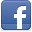Privacy Policy - Site Map - About Us - Letters to the Editor

Comments and inquiries could be addressed to:
webmaster@julianTrubin.comLast updated: June 2013
Copyright © 2003-2013 Julian Rubin